JEE  >  Test: 35 Year JEE Previous Year Questions: Circle

# Test: 35 Year JEE Previous Year Questions: Circle

Test Description

## 29 Questions MCQ Test Additional Documents and Tests for JEE | Test: 35 Year JEE Previous Year Questions: Circle

Test: 35 Year JEE Previous Year Questions: Circle for JEE 2022 is part of Additional Documents and Tests for JEE preparation. The Test: 35 Year JEE Previous Year Questions: Circle questions and answers have been prepared according to the JEE exam syllabus.The Test: 35 Year JEE Previous Year Questions: Circle MCQs are made for JEE 2022 Exam. Find important definitions, questions, notes, meanings, examples, exercises, MCQs and online tests for Test: 35 Year JEE Previous Year Questions: Circle below.
Solutions of Test: 35 Year JEE Previous Year Questions: Circle questions in English are available as part of our Additional Documents and Tests for JEE for JEE & Test: 35 Year JEE Previous Year Questions: Circle solutions in Hindi for Additional Documents and Tests for JEE course. Download more important topics, notes, lectures and mock test series for JEE Exam by signing up for free. Attempt Test: 35 Year JEE Previous Year Questions: Circle | 29 questions in 60 minutes | Mock test for JEE preparation | Free important questions MCQ to study Additional Documents and Tests for JEE for JEE Exam | Download free PDF with solutions
 1 Crore+ students have signed up on EduRev. Have you?
Test: 35 Year JEE Previous Year Questions: Circle - Question 1

### If the chord y = mx + 1 of the circle x2+y2=1 subtends an angle of measure 45° at the major segment of the circle then value of m is 

Detailed Solution for Test: 35 Year JEE Previous Year Questions: Circle - Question 1

Equation of circle x2 + y2 = 1 = (1)2
⇒ x2 + y2 = (y – mx)2 ⇒ x2 = m2x2 – 2 mxy;
⇒ x2 (1 – m2) + 2mxy = 0.
Which represents the pair of lines between which the angle is 45o.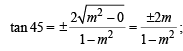⇒ 1 – m2 = ± 2m ⇒ m2 ± 2m – 1 = 0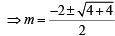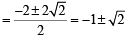Test: 35 Year JEE Previous Year Questions: Circle - Question 2

### The centres of a set of circles, each of radius 3, lie on the circle x2+y2=25. The locus of any point in the set is

Detailed Solution for Test: 35 Year JEE Previous Year Questions: Circle - Question 2

For any point P (x, y) in the given circle,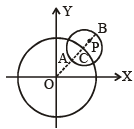we should have

OA ≤ OP ≤ OB ⇒ (5-3)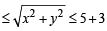⇒ 4 ≤ x2 +y2 ≤ 64

Test: 35 Year JEE Previous Year Questions: Circle - Question 3

### The centre of the circle passing through (0, 0) and (1, 0) and touching the circle x2+y2=9 is 

Detailed Solution for Test: 35 Year JEE Previous Year Questions: Circle - Question 3

Let the required circle be x2  +  y2 + 2gx  + 2fy + c = 0
Since it passes through (0, 0) and (1, 0)

⇒ c = 0 and  g = -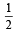Points (0, 0) and (1, 0) lie inside the circle x2 + y2 = 9, so two circles touch internally
⇒ c1c2 = r1 – r2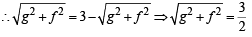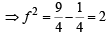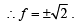Hence, the centres of required circle are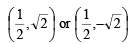Test: 35 Year JEE Previous Year Questions: Circle - Question 4

The equation of a circle with origin as a centre and passing through equilateral triangle whose median is of length 3a is

Detailed Solution for Test: 35 Year JEE Previous Year Questions: Circle - Question 4

Let ABC be an equilateral triangle, whose median is AD.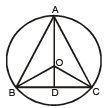In Δ ABD, AB2 = AD2 + BD2 ; ⇒ x2 = 9a2 + (x2/4)

where AB = BC = AC = x.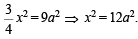In Δ OBD, OB2 = OD2 + BD2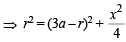⇒ r2 = 9a2 – 6ar + r2 + 3a2 ;
⇒ 6ar = 12a2 ⇒ r = 2a
So equation of circle is x2 + y2 = 4a2

Test: 35 Year JEE Previous Year Questions: Circle - Question 5

If the two circles ( x - 1)2 + ( y - 3)2 = r2 and x2 + y2 - 8 x + 2 y + 8 = 0 intersect in two distinct point, then

Detailed Solution for Test: 35 Year JEE Previous Year Questions: Circle - Question 5

r1 - r2< C1C2 for intersection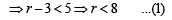and ,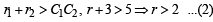From (1) and (2),  2 < r < 8.

Test: 35 Year JEE Previous Year Questions: Circle - Question 6

The lines 2 x - 3y=5 and 3x - 4 y = 7 are diameters of a circle having area as 154 sq.units.Then the equation  of the circle is

Detailed Solution for Test: 35 Year JEE Previous Year Questions: Circle - Question 6

πr2 = 154 ⇒r= 7
For centre on solving equation 2x -3y = 5& 3x -4y= 7
we get x = 1,y =-1
∴ centre = (1, –1 )
Equation of circle, ( x - 1)2 + (y + 1)2
=72 x2 + y2 -2x+2y= 47

Test: 35 Year JEE Previous Year Questions: Circle - Question 7

If a circle passes through the point (a, b) and cuts the circle x2 + y2 = 4 orthogonally, then the locus of its centre is

Detailed Solution for Test: 35 Year JEE Previous Year Questions: Circle - Question 7

Let the variable circle is x2 + y2 + 2gx + 2fy + c = 0 ......(1)
It passes through (a, b)
∴ a2 + b2 + 2 ga + 2 fb + c = 0 ......(2)

(1) cuts x2 +y2= 4 orthogonally

∴2(gx0+ f x0) = c-4 ⇒ c=4

∴ from (2) a2 + b2 + 2ga + 2fb + 4=0

∴  Locus of centre (–g,–f) is a2 + b2 - 2ax -2by +4= 0
or 2ax + 2by = a2 + b+ 4

Test: 35 Year JEE Previous Year Questions: Circle - Question 8

A variable circle passes through the fixed point A( p,q) and touches x-axis . The locus of the other end of the diameter through A is 

Detailed Solution for Test: 35 Year JEE Previous Year Questions: Circle - Question 8

Let the variable circle be x2 + y2 + 2gx + 2fy + c = 0 ....(1)

∴ p2 + q2 + 2gp + 2fq + c =0 ....(2)
Circle (1) touches x-axis,
∴ g2 - c = 0 ⇒ c=g2 .
From (2) p2 + q2 + 2gp + 2fq +g=0 ....(3)
Let the other end of diameter through (p, q) be (h, k),

then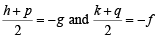Put in (3)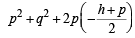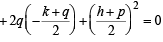⇒ h2 + p2 - 2hp - 4kq=0

∴ locus of (h, k) is x2 + p2 - 2 xp - 4 yq=0

⇒ ( x - p )2=4qy

Test: 35 Year JEE Previous Year Questions: Circle - Question 9

If the lines 2x + 3 y + 1 = 0 and 3x -y - 4=0 lie alon g diameter of a circle of circumference 10p, then the equation of the circle is 

Detailed Solution for Test: 35 Year JEE Previous Year Questions: Circle - Question 9

Two diameters are along 2x + 3y +1 = 0 and 3x -y - 4=0
solving we get centre (1, –1) circumference = 2πr = 10π
∴ r= 5.
Required circle is, ( x - 1)2 + (y + 1)2=52
⇒ x2 + y2 -2x+ 2y -23=0

Test: 35 Year JEE Previous Year Questions: Circle - Question 10

Intercept on the line y = x by the circle x2 + y2 - 2x = 0 is AB. Equation of the circle on AB as a diameter is 

Detailed Solution for Test: 35 Year JEE Previous Year Questions: Circle - Question 10

Solving y = x and the circle x2 + y2 - 2x= 0,
we get x = 0, y = 0 and x = 1,y=1
∴ Extremities of diameter of the required circle are (0, 0) and (1, 1).
Hence, the equation of circle is (x - 0)(x -1) + (y - 0)(y -1)= 0
⇒ x2 + y2 - x -y= 0

Test: 35 Year JEE Previous Year Questions: Circle - Question 11

If th e circles x2 + y2 + 2ax + cy + a = 0 and x2 + y2 – 3ax + dy – 1 = 0 intersect in two distinct points P and Q then the line 5x + by – a = 0 passes through P and Q for

Detailed Solution for Test: 35 Year JEE Previous Year Questions: Circle - Question 11

s1 = x2 + y2+ 2ax + cy +a =0
s2 = x+ y- 3ax + dy - 1 =0
Equation of common chord of circles s1 and s2 is given by s1 - s2= 0
⇒ 5ax + (c - d)y +a +1=0
Given that 5x + by – a = 0
passes through P and Q

∴ The two equations should represent the same line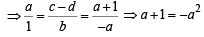a2 +a+1=0

No real value of a.

Test: 35 Year JEE Previous Year Questions: Circle - Question 12

A circle touches the x- axis and also touches the circle with centre at (0,3 ) and radius 2. The locus of the centre of the circle is

Detailed Solution for Test: 35 Year JEE Previous Year Questions: Circle - Question 12

Equation of circle with centre (0, 3) and radius  2 is x2 + (y - 3)2 = 4

Let locus of the variable circle is (α , β)

∵ It touches x - axis.

∴ It's equation is ( x - α)2 + (y + β)2 = β2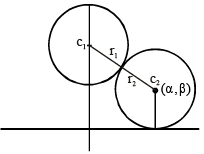Circle touch externally ⇒ c1c2 = r+ r2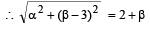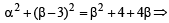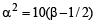∴ Locus is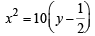which is a parabola.

Test: 35 Year JEE Previous Year Questions: Circle - Question 13

If a circle passes through the point (a, b) and cuts the circle x2 + y2 = p2 orthogonally, then the equation of the locus of its centre is 

Detailed Solution for Test: 35 Year JEE Previous Year Questions: Circle - Question 13

Let the centre be (α, β)

∵ It cuts the circle x2 + y= p2 orthogonally
∴ Using 2 g1 g2 +2 f1 f2 = c1+c2 , we get
2(-α) x 0 + 2(-β) x 0 = c1- p2 ⇒ c1 = p2
Let equation of circle is x2 + y2 - 2αx- 2βy+p2 =0
It passes through (a, b) ⇒ a2 + b2 - 2αa - 2βb +p2=0
∴Locus of (α, β) is
∴2ax+ 2by - (a2 +b2 + p2)=0 .

Test: 35 Year JEE Previous Year Questions: Circle - Question 14

If the pair of lines ax2 + 2 (a + b)xy + by 2 = 0 lie along diameters of a circle and divide the circle into four sectors such that the area of one of the sectors is thrice the area of another sector then 

Detailed Solution for Test: 35 Year JEE Previous Year Questions: Circle - Question 14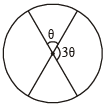As per question area of one sector = 3 area of another sector
⇒ angle at centre by one sector = 3x angle at centre by another sector
Let one angle be θ then other = 3θ
Clearly θ + 3θ  = 180 ⇒ θ = 45o

∴ Angle between the diameters represented by combined equation

ax2 + 2 ( a + b ) xy + by= 0 is 45o

∴ Using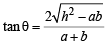we get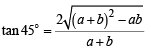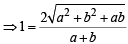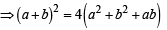⇒ a2 + b2 + 2ab = 4a2 + 4b+4ab ⇒ 3a+ 3b2 + 2ab = 0

Test: 35 Year JEE Previous Year Questions: Circle - Question 15

If the lines 3x - 4y - 7=0 and 2x - 3y - 5=0 are two diameters of a circle of area 49π square units, the equation of the circle is 

Detailed Solution for Test: 35 Year JEE Previous Year Questions: Circle - Question 15

Point of intersection of 3x - 4y - 7=0 and 2 x - 3y - 5=0 is (1, - 1)

which is the centre of the circle and radius = 7

∴ Equation is ( x - 1)2 + (y + 1)2= 49

⇒ x2 + y2 -2x+ 2y-47=0

Test: 35 Year JEE Previous Year Questions: Circle - Question 16

Let C be the circle with centre (0, 0) and radius 3 units. The equation of the locus of the mid points of the chords of the circle C that subtend an angle  of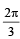at its center is

Detailed Solution for Test: 35 Year JEE Previous Year Questions: Circle - Question 16

Let M(h, k) be the mid point of chord AB where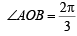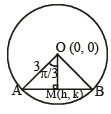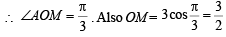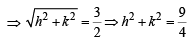∴ Locus of (h, k) is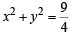Test: 35 Year JEE Previous Year Questions: Circle - Question 17

Consider a family of circles which are passing through the point (– 1, 1) and are tangent to x-axis. If (h, k) are the coordinate of the centre of the circles, then the set of values of k is given by the interval 

Detailed Solution for Test: 35 Year JEE Previous Year Questions: Circle - Question 17

Equation of circle whose centre is (h, k)

i.e (x – h)2 + (y – k)2 = k2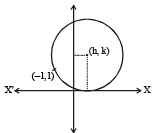(radius of circle = k because circle is tangent to x-axis) Equation of circle passing through (–1, +1)

∴ (–1 –h)2 + (1 – k)2 = k2

⇒ 1 + h2 + 2h + 1 + k2 – 2k = k2

⇒ h2 + 2h – 2k + 2 = 0 D ≥ 0
∴ (2)2 – 4 × 1.(–2k + 2) ≥ 0 ⇒ 4 – 4(–2k + 2) ≥ 0 ⇒ 1 + 2k – 2 ≥ 0 ⇒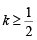Test: 35 Year JEE Previous Year Questions: Circle - Question 18

The point diametrically opposite to the point P(1, 0) on the circle x2 + y2 + 2x + 4y – 3 = 0 is 

Detailed Solution for Test: 35 Year JEE Previous Year Questions: Circle - Question 18

The given circle is  x2 + y2 + 2x + 4y –3 = 0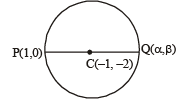Centre (–1, –2)

Let Q (α, β) be the point diametrically opposite to the point P(1, 0),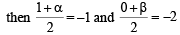⇒ α = –3, β = – 4, So, Q is (–3, –4)

Test: 35 Year JEE Previous Year Questions: Circle - Question 19

The differential equation of the family of circles with fixed radius 5 units and centre on the line y = 2 is

Detailed Solution for Test: 35 Year JEE Previous Year Questions: Circle - Question 19

Let the centre of the circle be (h, 2)

∴ Equation of circle is ( x– h)2 + (y – 2)2 = 25 …(1)

Differentiating with respect to x, we get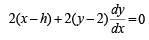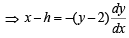Substituting in equation (1) we get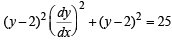⇒ (y – 2)2  (y')2 = 25 –  (y –2)2

Test: 35 Year JEE Previous Year Questions: Circle - Question 20

If P an d Q are the points of intersection of the circles x2 + y2 + 3x + 7y+ 2p- 5 =0 and x+ y2 + 2x + 2y – p= 0 then there is a circle passing through P, Q and (1, 1) for: 

Detailed Solution for Test: 35 Year JEE Previous Year Questions: Circle - Question 20

The given circles are S1 ≡ x2 + y2 + 3x + 7y + 2p  – 5 = 0 ....(1)
S2 ≡ x2 + y2 + 2x + 2y – p2 = 0 ....(2)
∴ Equation of common chord PQ is S1 – S2 = 0

⇒ L≡ x+5y+ p2 + 2p -5=0
⇒ Equation of circle passing through P and Q is S1 + λL = 0
⇒ (x2 + y2 + 3x + 7y + 2p  – 5) + λ (x + 5y + p2 +2p – 5) = 0
As it passes through (1, 1), therefore

⇒ (7 + 2p ) + λ (2p + p2 + 1) = 0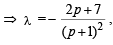which does not exist for p = – 1

Test: 35 Year JEE Previous Year Questions: Circle - Question 21

The circle x2 + y2 = 4x + 8y + 5 intersects the line 3x – 4y = m at two distinct points if 

Detailed Solution for Test: 35 Year JEE Previous Year Questions: Circle - Question 21

Circle x2 + y2 - 4 x - 8y - 5=0

Centre = (2, 4), Radius =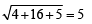If circle is intersecting line 3x - 4 y=m, at two distinct points.
⇒ length of perpendicular from centre to the line < radius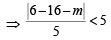⇒ |10 + m| < 25

⇒ –25 < m  + 10 < 25 ⇒ – 35 < m < 15

Test: 35 Year JEE Previous Year Questions: Circle - Question 22

The two circles x2 + y2 = ax and x2 + y2 = c2 (c > 0) touch each other if 

Detailed Solution for Test: 35 Year JEE Previous Year Questions: Circle - Question 22

As centre of one circle is (0, 0) and other circle passes through (0, 0), therefore

Also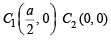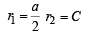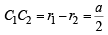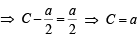If the two circles touch each other, then they must touch each other internally.

Test: 35 Year JEE Previous Year Questions: Circle - Question 23

The length of the diameter of the circle which touches the x-axis at the point (1,0) and passes through the point (2,3) is: 

Detailed Solution for Test: 35 Year JEE Previous Year Questions: Circle - Question 23

Let centre of the circle be (1,h)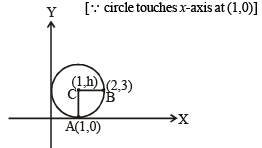Let the circle passes through the point B (2,3)

∴ CA = CB (radius) ⇒ CA2 = CB2
⇒ (1 – 1)2 + (h  – 0)2 = (1 – 2)2 + ( h – 3)2

⇒ h2 = 1 + h2 + 9 – 6h ⇒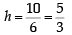Thus, diameter is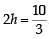Test: 35 Year JEE Previous Year Questions: Circle - Question 24

The circle passing through (1, –2) and touching the axis of x at (3, 0) also passes through the point [JEE M 2013]

Detailed Solution for Test: 35 Year JEE Previous Year Questions: Circle - Question 24

Since circle touches x-axis at (3, 0)

∴ The equation of circle be (x – 3)2 + (y – 0)2 + λy = 0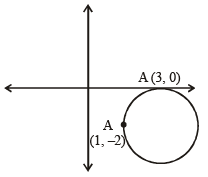As it passes through (1, –2)

∴ Put x = 1, y = –2

⇒ (1 – 3)2 + (–2)2 + λ(–2) = 0 ⇒ λ = 4

∴ equation of circle is (x – 3)2 + y2 – 8 = 0

Now,  from the options (5, –2) satisfies equation of circle.

Test: 35 Year JEE Previous Year Questions: Circle - Question 25

Let C be the circle with centre at (1, 1) and radius = 1. If T is the circle centred at (0, y), passing through origin and touching the circle C externally, then the radius of T is equal to [JEE M 2014]

Detailed Solution for Test: 35 Year JEE Previous Year Questions: Circle - Question 25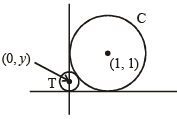Equation of circle C ≡ ( x- 1)2 + (y- 1)2 =1

Radius of T = | y |
T touches C externally therefore,
Distance between the centres = sum of their radii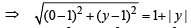⇒ (0 – 1)2 + (y –1)2 = (1 + |y|)2
⇒ 1 + y2 + 1 – 2y = 1 + y2 + 2| y |
2 | y | = 1 – 2y

If y > 0 then 2y = 1 – 2y ⇒ y  =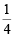If y < 0 then –2y = 1 – 2y ⇒ 0 = 1 (not possible)

∴ y =Test: 35 Year JEE Previous Year Questions: Circle - Question 26

Locus of the image of the point (2, 3) in the line (2x – 3y + 4) + k (x – 2y + 3) = 0, k ∈ R, is a : [JEE M 2015]

Detailed Solution for Test: 35 Year JEE Previous Year Questions: Circle - Question 26

Intersection point of 2x – 3y + 4 = 0 and x – 2y + 3 = 0 is (1, 2)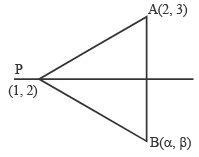Since, P is the fixed point for given family of lines So, PB = PA
(α – 1)2 + (β – 2)2 = (2 – 1)2 + (3 – 2)2
(α – 1)2 + (β –2)2 = 1 + 1 = 2
(x – 1)2 + (y – 2)2 =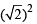(x – α)2 + (y – β)2 = r2
Therefore, given locus is a circle with centre (1, 2) and radius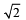Test: 35 Year JEE Previous Year Questions: Circle - Question 27

The number of common tangents to the circles x2 + y2 – 4x  – 6x – 12 = 0 and x2 + y2 + 6x + 18y + 26 = 0, is   :[JEE M 2015]

Detailed Solution for Test: 35 Year JEE Previous Year Questions: Circle - Question 27

x2 + y2 – 4x – 6y – 12 = 0 ...(i)
Centre, c1 = (2, 3) and
Radius, r1 = 5 units x2 + y2 + 6x + 18y + 26 = 0 ...(ii)
Centre, c2 = (–3, –9) and Radius, r2 = 8 units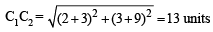r1 + r2 = 5 + 8 = 13

∴ C1 C2 = r1 + r2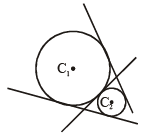Therefore there are three common tangents.

Test: 35 Year JEE Previous Year Questions: Circle - Question 28

The centres of those circles which touch the circle,  x2 + y2 – 8x – 8y – 4 = 0, externally and also touch the x-axis,lie on: [JEE M 2016]

Detailed Solution for Test: 35 Year JEE Previous Year Questions: Circle - Question 28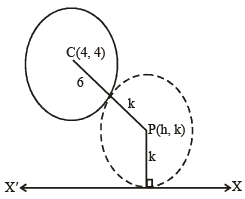For the given circle, centre : (4, 4) radius = 6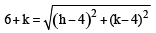(h – 4)2 = 20k + 20
∴ locus of (h, k) is (x – 4)2 = 20(y + 1), which is a parabola.

Test: 35 Year JEE Previous Year Questions: Circle - Question 29

If one of the diameters of the circle, given by the equation, x2 + y2 – 4x + 6y – 12 = 0, is a chord of a circle S, whose centre is at (–3, 2), then the radius of S is: [JEE M 2016]

Detailed Solution for Test: 35 Year JEE Previous Year Questions: Circle - Question 29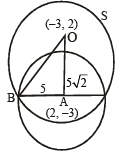Centre of S : O (–3, 2) centre of given circle A(2, –3)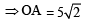Also AB = 5 (∵ AB = r of the given circle)

⇒ Using pythagoras theorem in ΔOAB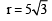## Additional Documents and Tests for JEE

11 videos|159 docs|14 tests
 Use Code STAYHOME200 and get INR 200 additional OFF Use Coupon Code
Information about Test: 35 Year JEE Previous Year Questions: Circle Page
In this test you can find the Exam questions for Test: 35 Year JEE Previous Year Questions: Circle solved & explained in the simplest way possible. Besides giving Questions and answers for Test: 35 Year JEE Previous Year Questions: Circle, EduRev gives you an ample number of Online tests for practice

## Additional Documents and Tests for JEE

11 videos|159 docs|14 tests